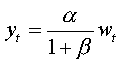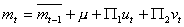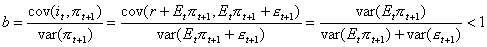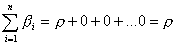# Prof. Bryan Caplan

bcaplan@gmu.edu

http://www.gmu.edu/departments/economics/bcaplan

Econ 816

Spring, 2000

Part 1: Mathematical Problems

1.  If mt is constant, then the money supply shocks all disappear.  Just set the variance of the money shock equal to zero!  Therefore, using the results from the notes:andIntuitively, getting rid of monetary instability gets rid of the economic shocks associated with monetary uncertainty.

2.AND.

Main intuition: To raise the nominal interest rate by 1%, you need to permanently raise the expected rate of money growth by 1%.  Notice that even when money has no real effects, the central bank could permanently raise nominal interest rates in this manner!

Secondary intuition: To actually hit the pegged rate, you have to adjust the rate of money growth on a period-by-period basis.  But since shocks are transitory, your expected money supply in any period is independent of past shocks.

3. Romer, problem 9.4.

a.  Your hypothesis is that: it=r+Etpt+1, with r constant.

Imposing rational expectations, pt+1=Etpt+1+et+1.

Regressing i on a constant and pt+1, so it=a+bpt+1+et:So b, your best estimate for b, is less than 1.

b. pt+1=a+bit+ut; estimating b:c.  Inflation assumed to be white noise process: pt=rpt+et.

Regressing it on many lags of inflation:With constant real interest rate, nominal rates move 1:1 with expected inflation.  However, if inflation is a white noise process, it does not follow that the betas sum to one.  In fact, the white noise assumption implies Etpt=rpt-1, so:

b0=r, and all of the other betas =0.  Therefore,, which is not necessarily equal to 1.

Part 2: Analytical Problems

I attach sample answers from Eric Crampton for the first two questions, along with some general and question-specific comments.

1.  When do deviations from "rational expectations in the aggregate" occur?  When do severe instances of the "bias towards zero in aggregate perceptions" appear?  Is there any common pattern that both deviations from RE share?

Many people didn't seem to understand the distinction between:

i.  rational expectations

ii.  rational expectations in the aggregate

iii.  DEVIATIONS from rational expectations in the aggregate

Haltiwanger and Waldman explored (ii).  As they explained, (i) is a special case of (ii).  If everyone has RE, then RE in the aggregate has to hold; but just because RE holds in the aggregate, it does not follow that everyone has RE.

Moreover, the question asked about (iii), not (ii).  Many people summarized the H&W article, which you were not asked to do.  Few if any people mentioned the most obvious connection between deviations "RE in the aggregate" and "the bias towards zero": the latter is a special case of the former!

Last, many people said that e.g. "Synergy causes RE in the aggregate."  No.  RE in the aggregate (as opposed to regular RE) matters more when there is synergy, but synergy does not cause RE in the aggregate.

2.  Agree or disagree: Caplan's "rational irrationality" analysis suggests that the rational expectations assumption probably applies in all macroeconomic models so long as they do not have a political component.

Most people just summarized the main idea of the paper.  The good answers usually disagreed, noting that it is possible to have a macro model without politics with small private error costs (for example, Akerlof and Yellen's model of second-order small deviations from rationality).

3.  Pick a specific government policy that you think is inefficient.  Which, if any, of Caplan's findings in "Systematically Biased Beliefs About Economics," would explain the existence of this policy?  Do Caplan's findings make "special interest"/rent-seeking explanations of your example superfluous?